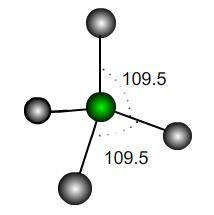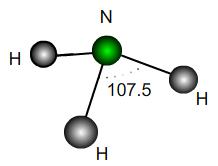#### You may also likeA quadrilateral changes shape with the edge lengths constant. Show the scalar product of the diagonals is constant. If the diagonals are perpendicular in one position are they always perpendicular?As a quadrilateral Q is deformed (keeping the edge lengths constnt) the diagonals and the angle X between them change. Prove that the area of Q is proportional to tanX.### Air Routes

Find the distance of the shortest air route at an altitude of 6000 metres between London and Cape Town given the latitudes and longitudes. A simple application of scalar products of vectors.

# Bond Angles

##### Age 16 to 18Challenge Level

This problem makes use of vectors, coordinates and scalar products. It is a very useful fact that if two vectors ${\bf u}$ and ${\bf v}$ have coordinates $(x_1, y_1, z_1)$ and $(x_2, y_2, z_2)$ then the cosine of the angle $\theta$ they make is$$\cos(\theta) = \frac{{\bf u}\cdot {\bf v}}{|{\bf u}||{\bf v}|}\quad\,,\mbox{ where } {\bf u}\cdot {\bf v}=x_1x_2+y_1y_2+z_1z_2$$
This is useful, because if we can label the coordinates of the atoms in a molecule or crystal then we can easily work out the angles between the bonds.

 In a perfect tetrahedral molecule there is a central atom attached to four other atoms which lie on the vertices of a perfect tetrahedron. If the central atom is at the coordinate origin and the molecule rests on the plane $z=-h$ then what would be the coordinates of the other atoms, assuming a bond length of $1$ unit? Find the scalar products between the vectors joining the origin to each atom and hence the value of $h$. It is usually stated that the angles each of these bonds make is $109.5^\circ$; however, this is only an approximation. What is the exact value of the bond angle in a perfect tetrahedron? If the perfect tetrahedron is deformed slightly, how many of the bond angles could be exactly $109.5^\circ$? How would it be deformed to achieve this? Would the other bond angles increase or decrease under such a deformation?A perfect trigonal pyramidal molecule is the same as a perfect tetrahedral molecule with a single outer atom removed. Ammonia NH$_3$ is approximately a trigonal pyramid with bond angle $107.5$. Does this correspond to a lengthening or shortening of the bonds relative to a perfect structure? By what percentage? If the H atoms were fixed and a vertical force were applied downwards to the N atom in the diagram, would the bond angles increase or decrease? What would be the maximum possible angle mathematically?